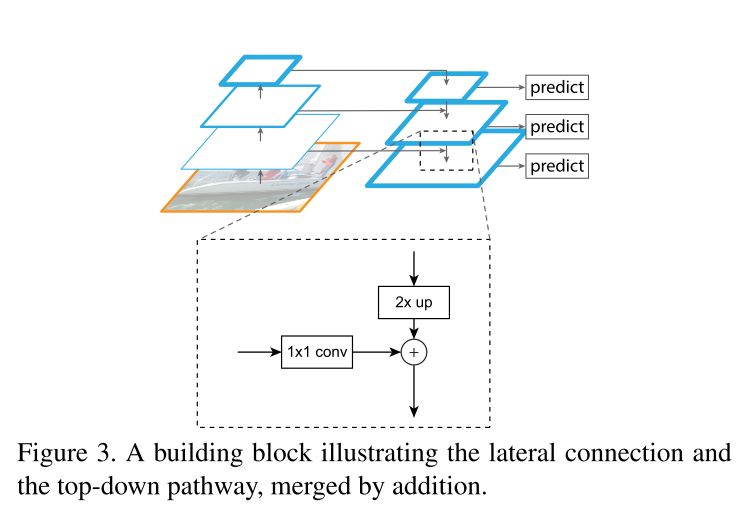# 1. 为什么会使用金字塔式的representation以及它存在的问题。

• 为了得到图像在不同尺寸下的金字塔式的表示，使用图像金字塔去生成对应的特征金字塔在理论上虽然是可行，但是特征金子塔的推理时间相当的长，在追求高效和实时的需求下的劣势非常明显。除此之外，如果想要配合深度学习进行端对端的训练，是不现实的，因为计算量过于巨大。
• 除了使用图像金字塔以外的方法，还可以使用卷积神经网络本身自带的金字塔属性（每一个卷积层输出的特征图的大小会逐渐变小，最后得到一些高语义的特征。）来构建一个 in-network feature hierarchy来产出不同大小的feature map.但是这样的作法也存在着问题，不同的深度会导致较大的semantic gap 从而影响到网络进行物体检测的表现。# 3. 如何基于resnet实现（思路）。

``````class Bottleneck(nn.Module):
expansion = 4

def __init__(self, in_planes, planes, stride=1):
super(Bottleneck, self).__init__()
self.conv1 = nn.Conv2d(in_planes, planes, kernel_size=1, bias=False)
self.bn1 = nn.BatchNorm2d(planes)
self.conv2 = nn.Conv2d(planes, planes, kernel_size=3, stride=stride, padding=1, bias=False)
self.bn2 = nn.BatchNorm2d(planes)
self.conv3 = nn.Conv2d(planes, self.expansion*planes, kernel_size=1, bias=False)
self.bn3 = nn.BatchNorm2d(self.expansion*planes)

self.shortcut = nn.Sequential()
if stride != 1 or in_planes != self.expansion*planes:
self.shortcut = nn.Sequential(
nn.Conv2d(in_planes, self.expansion * planes, kernel_size=1, stride=stride, bias=False),
nn.BatchNorm2d(self.expansion*planes)
)

def forward(self, x):
out = F.relu(self.bn1(self.conv1(x)))
out = F.relu(self.bn2(self.conv2(out)))
out = self.bn3(self.conv3(out))
out += self.shortcut(x)
out = F.relu(out)
return out

``````

``````  	class FPN(nn.Module):
def __init__(self, block, num_blocks):
super(FPN, self).__init__()
self.in_planes = 64

self.conv1 = nn.Conv2d(3, 64, kernel_size=7, stride=2, padding=3, bias=False)
self.bn1 = nn.BatchNorm2d(64)

# resnet forward propagation / Bottom-up pathway
self.layer1 = self._make_layer(block,  64, num_blocks, stride=1)
self.layer2 = self._make_layer(block, 128, num_blocks, stride=2)
self.layer3 = self._make_layer(block, 256, num_blocks, stride=2)
self.layer4 = self._make_layer(block, 512, num_blocks, stride=2)
``````

`````` self.top_layer = nn.Conv2d(2048, 256, kernel_size=1, stride=1, padding=0)
``````

`````` 		self.anti_alising_1 = nn.Conv2d(256, 256, kernel_size=3, stride=1, padding=1)
self.anti_alising_2 = nn.Conv2d(256, 256, kernel_size=3, stride=1, padding=1)
self.anti_alising_3 = nn.Conv2d(256, 256, kernel_size=3, stride=1, padding=1)
``````

``````		self.lateral_layer1 = nn.Conv2d(1024, 256, kernel_size=1, stride=1, padding=0)
self.lateral_layer2 = nn.Conv2d( 512, 256, kernel_size=1, stride=1, padding=0)
self.lateral_layer3 = nn.Conv2d( 256, 256, kernel_size=1, stride=1, padding=0)
``````

``````torch.Size([1, 256, 56, 56])
torch.Size([1, 256, 28, 28])
torch.Size([1, 256, 14, 14])
torch.Size([1, 256, 7, 7])
``````

# 4. 小总结

FPN利用了CNN自带的金子塔式的结构特点，使用了 bottom-up， top-down以及 lateral connection三个不同的路径，生成了具有高语义信息的特征图。这样技术可以被运用到了现在一些主流的物体检测和物体分割的网络中，比如mask rcnn。它有效的提高了网络的表现以及在一定的程度上解决了 pyramid representation的一些固有问题。